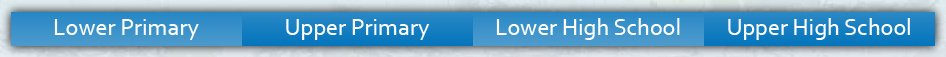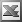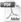Lower High School Enter keywords to search by topic or worksheet description

Worksheets in this category help students to read, write and understand decimals, and to understand their relationship to both common fractions and percentages.

Worksheet Name Files
Decimals: Place Value and Decimal AdditionDecimals: Comparing Using SymbolsRounding DecimalsDecimals: Estimating Decimal AdditionDecimals in Different Forms: Pictures, Numerals, WordsDecimals: Arranging in OrderDecimals in Different Forms: Numerals, Fractions, WordsRounding Decimals: INTERACTIVEDecimals: Writing in WordsDecimals: Place Value of Digits to ThousandthsTerminating and Recurring Decimals